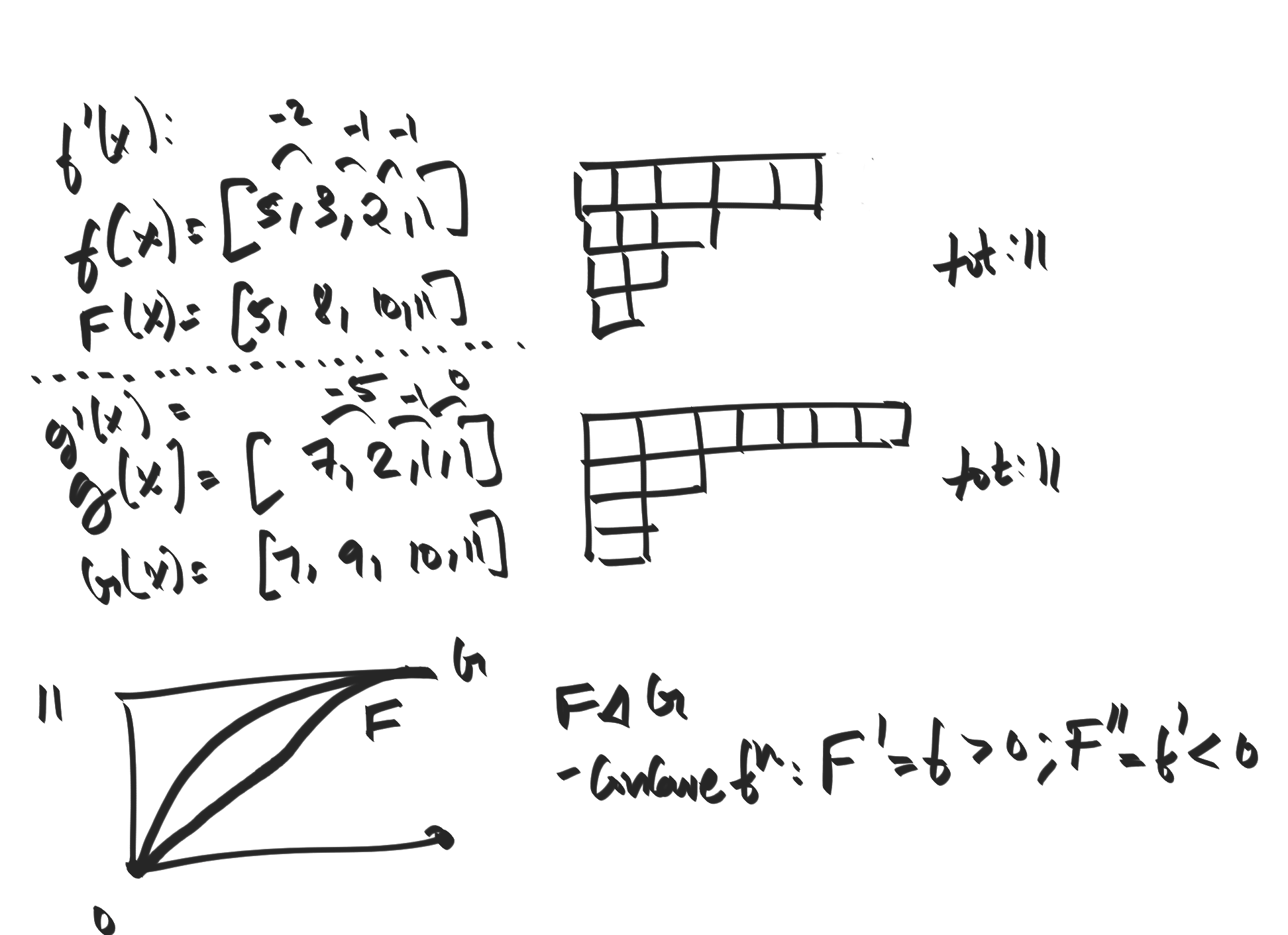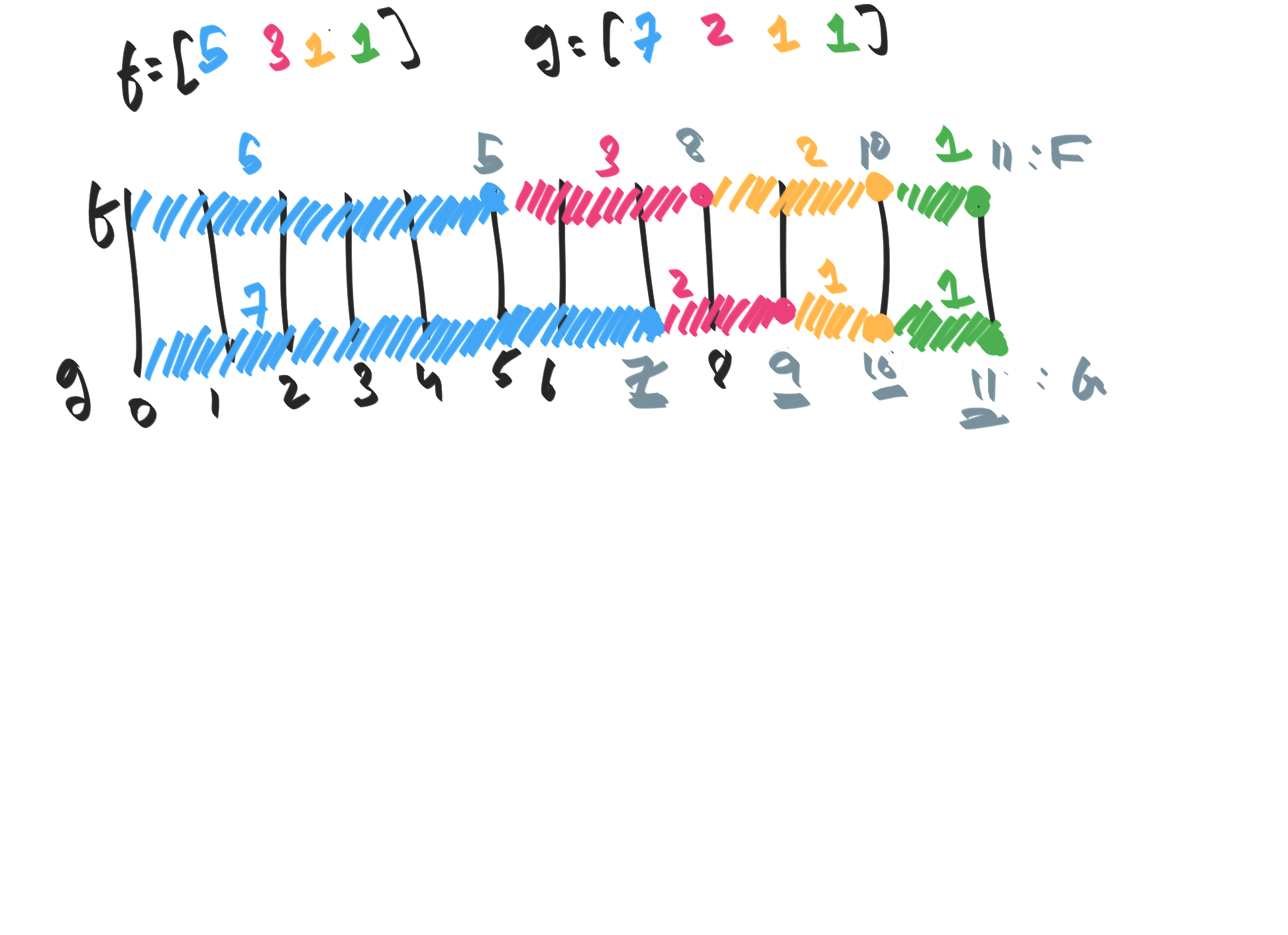We denote by $\sum_! F(x, \dots, x[n])$ the sum ov $F$ evaluated over all permutations. Formally:
$\sum_! F(x, \dots, x[n]) \equiv \sum_{\sigma \in S_n} F(x[\sigma], \dots, x[\sigma[n]])$
We write
$[a, \dots a[n]] \equiv 1/n! \sum_! x_1^{a} \dots x[n]^{a[n]} = \sum_{\sigma \in S_n} \prod_{j} x[\sigma[j]]^{a[j]}$
For example,we have:
• $[1, 1] = 1/2! (xy + yx) = xy$
• $[1, 1, 1] = xyz$
• $[2, 1, 0] = 1/3! (x^2y + x^2z + y^2z + y^2x + z^2x + z^2 y)$
• $[1, 0, 0] = 1/3! (x^{[1, 0, 0']} + x^{[1, 0', 0]} + x^{[0, 1, 0']} + x^{[0', 1, 0]} + x^{[0, 0', 1]} + x^{[0', 0, 1]})$. This is equal to $2!(x + y + z)/3! = (x + y + z)/3$.
• In general, $[1, 0, 0, 0, \dots, 0]$ ( $n$ zeroes) is $(n-1)!/n!(\sum_i x_i)$ which is the AM.
• Also, $[1/2, 1/2] = 1/2!(x^{1/2}y^{1/2} + y^{1/2}x^{1/2}) = \sqrt{xy}$.
• In general, $[1/n, 1/n, \dots, 1/n]$ is the GM $\sqrt{n}{x x \dots x[n]}$.

#### § Majorization

Let $(a), (b) \in \mathbb R^n$ be two non-decreasing sequences: $a \geq a \dots \geq a[n]$, and $b \geq b \dots \geq b[n]$. We will say that $(b)$ is majorized by $(a)$ (written as $(b) \prec (a)$) when we have that:
1. $a \geq a \geq \dots a[n]$, $b \geq b \geq \dots b[n]$.
2. $\sum_i b[i] = \sum_i a[i]$.
3. $\sum_{i=1}^u b[i] \leq \sum_{i=1}^u a[i]$ for $1 \leq i \leq n$.
It is clear that this is a partial order. The below figure shows majorization in terms of partitions. For two sequences $f, g$, define $F$ to be their "integral" (partial sums) and $f', g'$ to be their "derivatives" (finite differences). Then the condition that $f \prec g$ states that $F$ is upper bounded by $G$, and that $F, G$ are concave functions.The other lens of viewing majorization is to think of a number as some sort of fixed length $l$, and we are allowed to make some progress to reach the goal ( $f(x) > 0$) as long as we progress less at each each timestep ( $f''(x) < 0$). In this viewpoint, the majorization condition asserts that $f \prec g$ is that $g$ will always be ahead of/not fall behind $f$.#### § Majorization and step

We can show that if $(b) \prec (a)$ , then we can get from $(b)$ to $(a)$ in a finite number of discrete steps, that "borrow" from higherlocations in $b$ and "give" to lower locations. Formally, define a step operator $S(l, r)$ where $l < r$ such that:
$S(l, r)(b)[k] = \begin{cases} b[l]+1 & k = l \\ b[r]-1 & k = r \\ b[k] & \texttt{otherwise} \end{cases}$
That is, this borrows a single value from $b[j]$ and gives it to $b[i]$. We can see that $(b) \prec S(l, r)(b)$. For a given $(b) \prec (a)$, we can find a sequence of step operations $S(l[i], r[i])$ such that we transform $(b)$ into $(a)$; That is, it is possible to "single-step" the translation from $(b)$ to $(a)$.

for non negative numbers $x[]$, we have:
$[b] \leq [a] \iff (b) \prec (a)$
$1/n! \sum_! x^{[b]} \leq 1/n! \sum_! x^{[a]} \iff (b) \prec (a) \\ \sum_! x^{[b]} \leq \sum_! x^{[a]} \iff (b) \prec (a) \\$